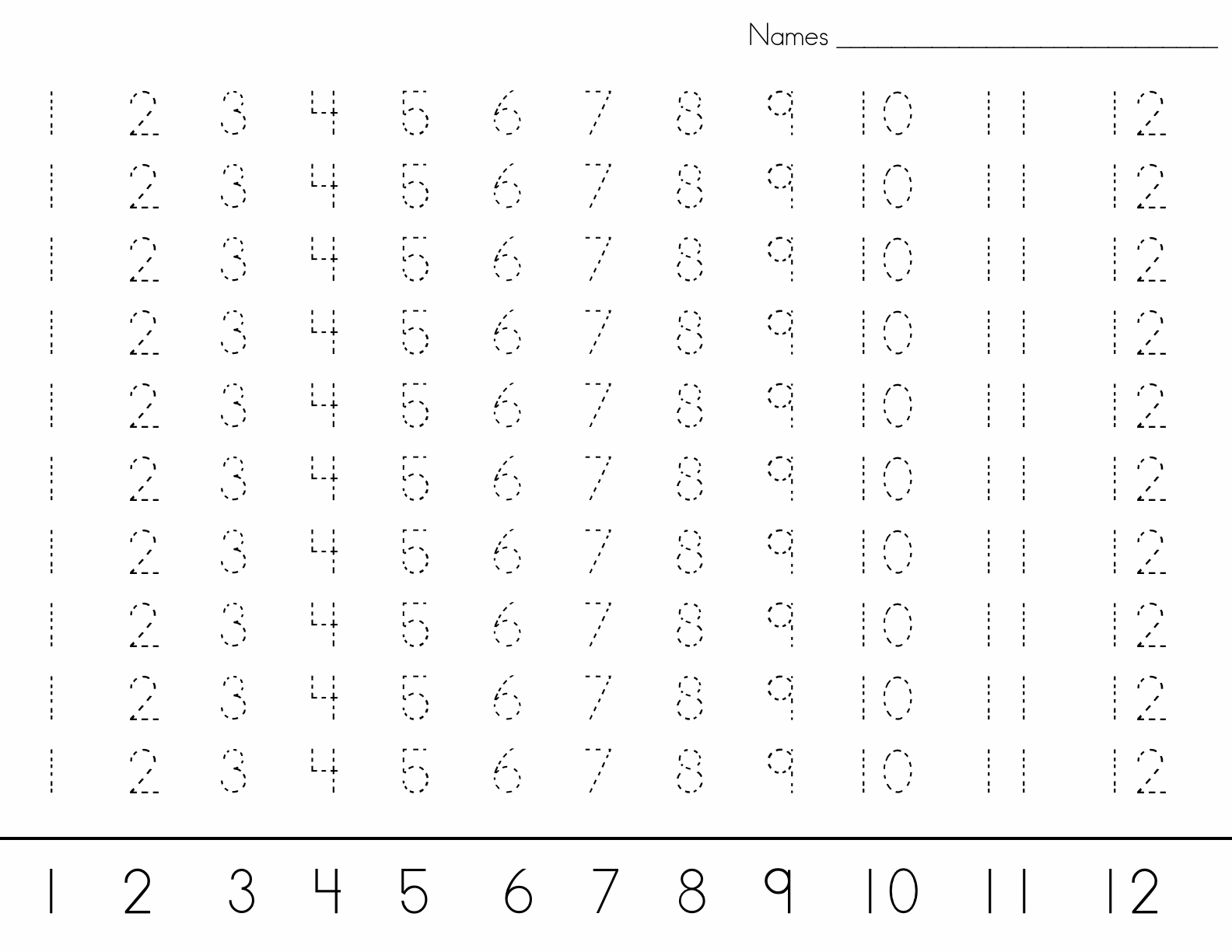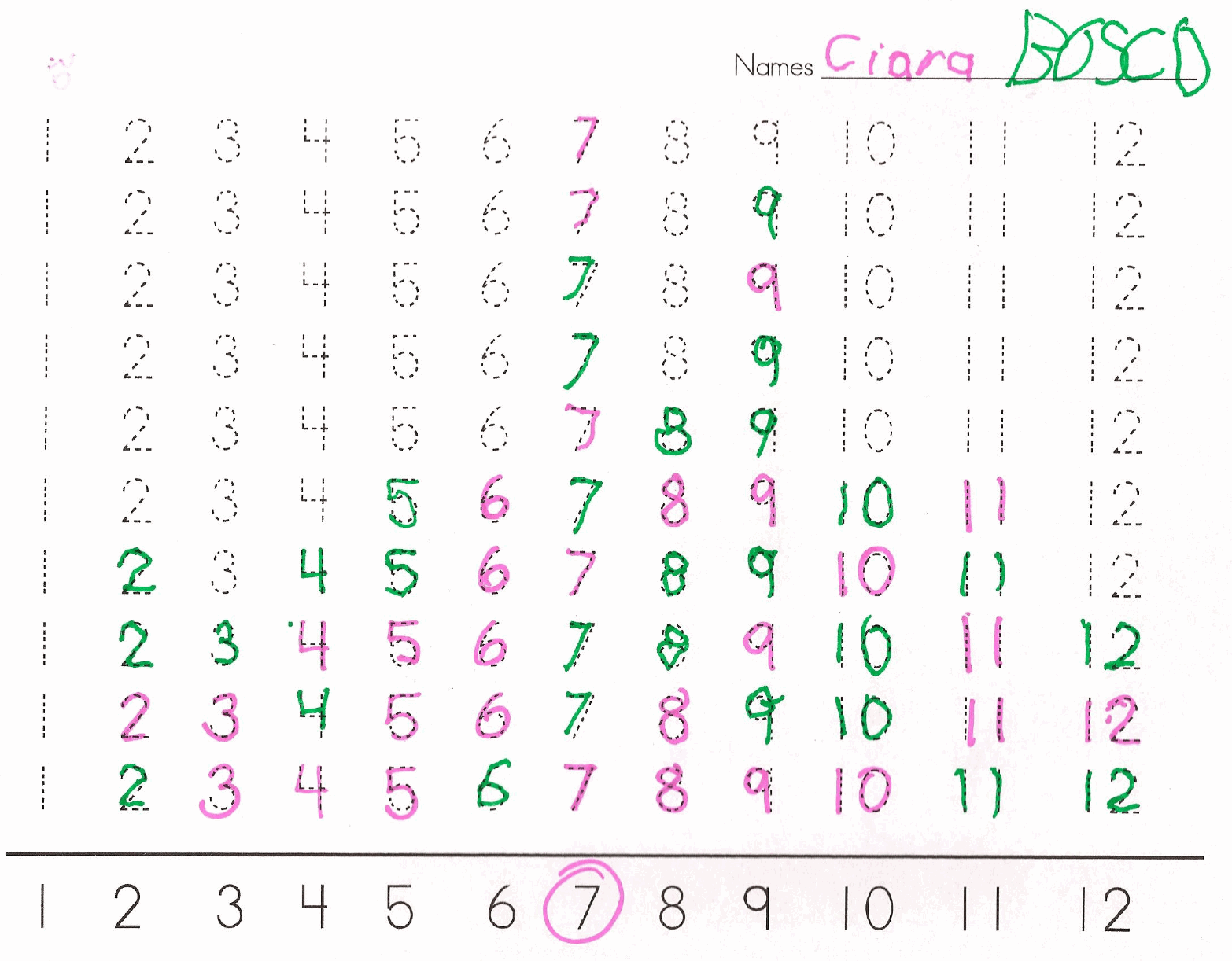##### Engage your students with effective distance learning resources. ACCESS RESOURCES>>

Alignments to Content Standards: K.CC.A.3 K.OA.A.2

#### Materials

Each pair of students needs: * One worksheet * Two markers of different colors * One pair of dice

#### Action

Student A rolls the two dice, finds the sum, and traces the number on the worksheet which corresponds to the answer with his/her marker. Student A then passes the dice to Student B who rolls both the dice, finds the sum and traces the correct number on the worksheet with his/her marker. Play continues this way until one of the numbers “wins” (i.e. all of the numbers of that quantity have been traced).## IM Commentary

This task supports students in correctly writing numbers. Because students have to trace the number instead of coloring in a bubble with the number in it or circling the correct number, they gain handwriting practice as well as counting and addition practice.

The standard for kindergarten is addition to 10. This game uses two commercially available six-sided dice, and because of this students will practice addition to 12. If a teacher feels his/her class is not ready for addition to 12 they can use two spinners of 1-5 (or even 0-5) or use non-standard dice which go 0-5 or 1-5.

The teacher can start this game with dot dice so that students can count the dots to determine the sum. If the teacher would like to differentiate for students that need more of a challenge s/he can change the dice so that one die has written numbers and once die has dots. This will encourage students to practice counting on. The teacher could also give students two dice with written numbers. Teachers can strategically give different students different types of dice as a way of differentiating. This activity is an excellent independent center which students can play from the time they have been introduced to addition until the end of the school year.

As an extension, after students have played this game several times, the teacher can take a few completed game boards and the class can talk about trends they see and why. For example, no one ever gets the sum of one, why is that? Also if many games boards are compared students will probably find that numbers 6, 7, 8 and 9 win more often then numbers 2 and 12. The class could discuss this finding and why they believe it happens.

As an extension to this task see K.OA Dice Addition 2, which deals with the same concept but has students write out the equations they create with the dice.

## Solution

There is no one solution for this task. Students have correctly solved the task when they have taken turns, counted the dots on their dice and recorded the sum until one number is the "winner."